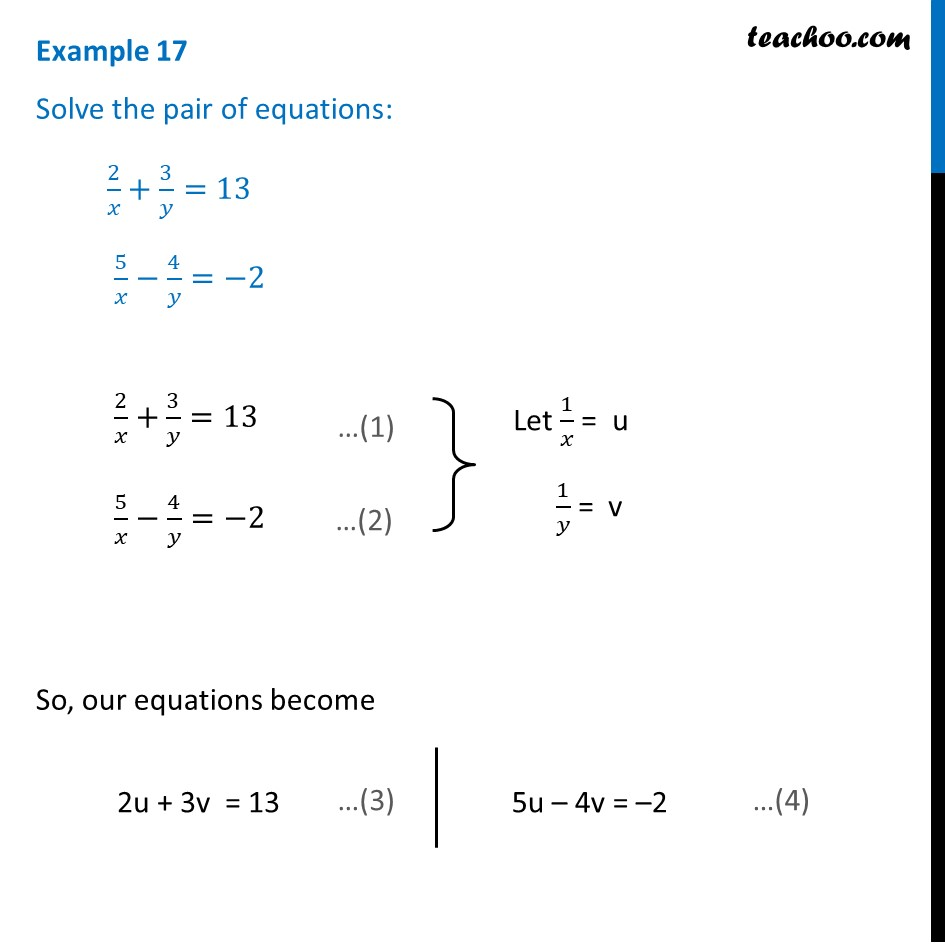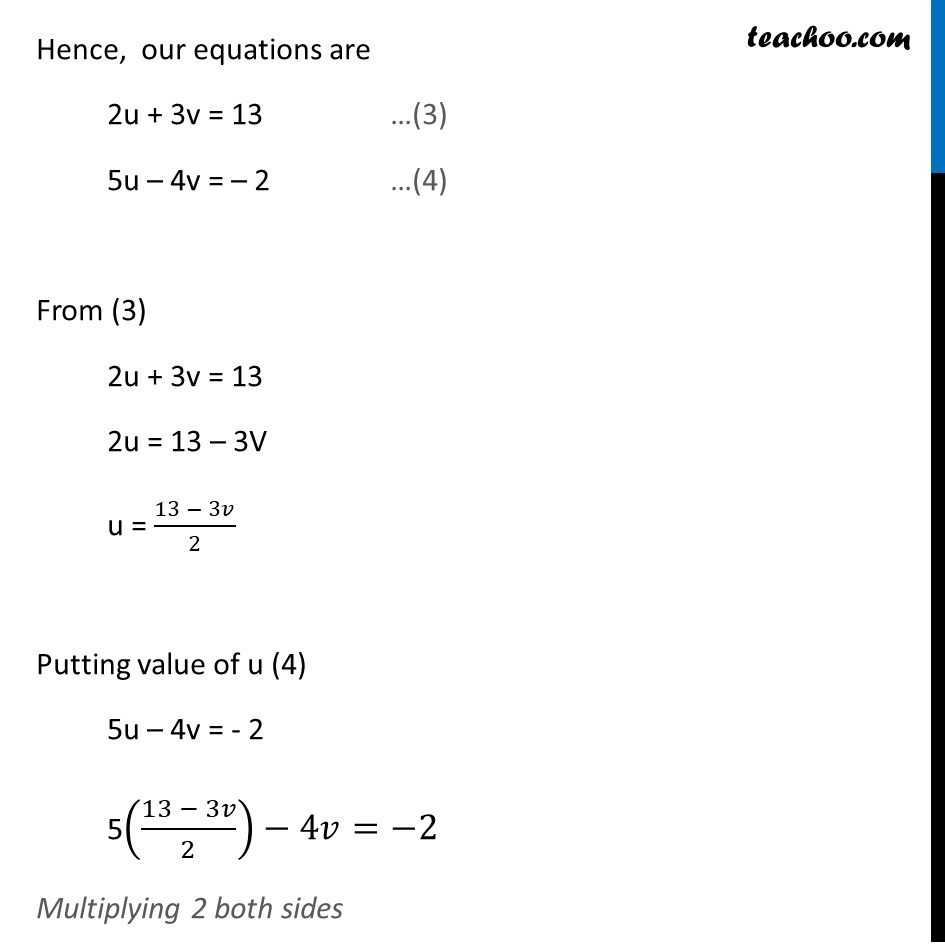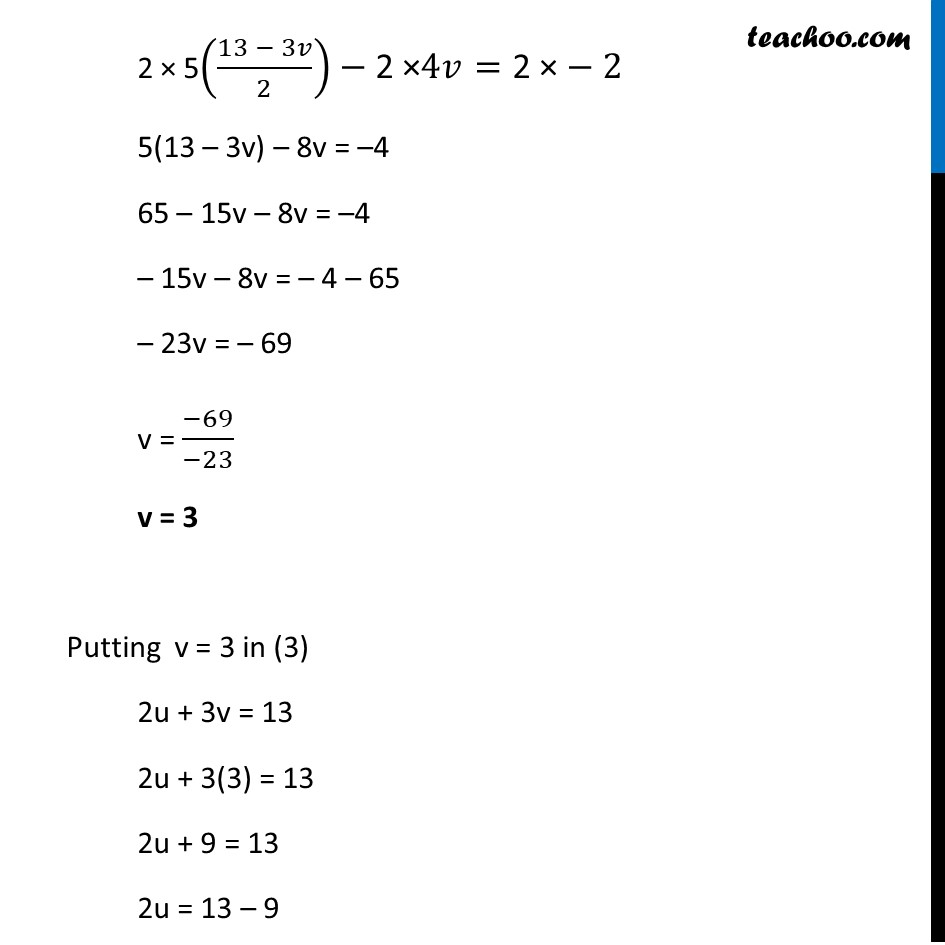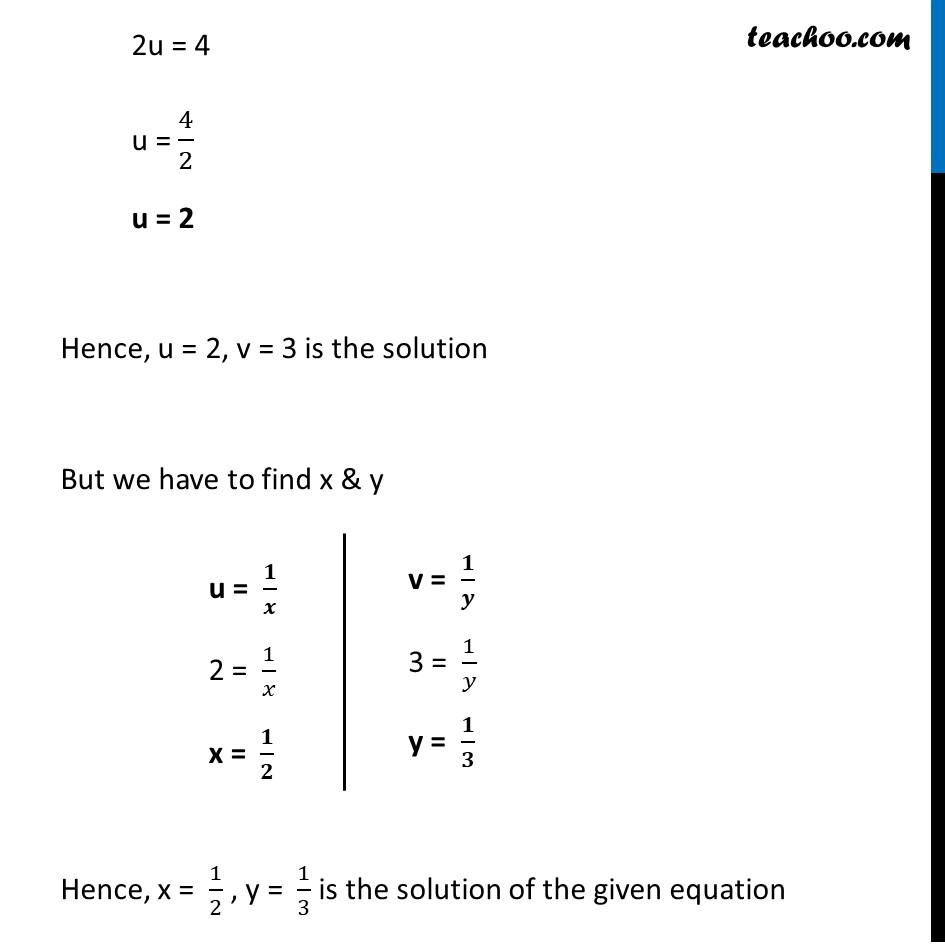1. Chapter 3 Class 10 Pair of Linear Equations in Two Variables (Term 1)
2. Concept wise
3. Mix questions - Equation given

Transcript

Example 17 Solve the pair of equations: 2/𝑥+ 3/𝑦=13 5/𝑥−4/𝑦=−2 2/𝑥+ 3/𝑦=13 5/𝑥−4/𝑦=−2 So, our equations become 2u + 3v = 13 5u – 4v = –2 Hence, our equations are 2u + 3v = 13 …(3) 5u – 4v = – 2 …(4) From (3) 2u + 3v = 13 2u = 13 – 3V u = (13 − 3𝑣)/2 Putting value of u (4) 5u – 4v = - 2 5((13 − 3𝑣)/2)−4𝑣=−2 Multiplying 2 both sides 2 × 5((13 − 3𝑣)/2)−"2 ×" 4𝑣="2 ×"−2 5(13 – 3v) – 8v = –4 65 – 15v – 8v = –4 – 15v – 8v = – 4 – 65 – 23v = – 69 v = (−69)/(−23) v = 3 Putting v = 3 in (3) 2u + 3v = 13 2u + 3(3) = 13 2u + 9 = 13 2u = 13 – 9 2u = 4 u = 4/2 u = 2 Hence, u = 2, v = 3 is the solution But we have to find x & y u = 𝟏/𝒙 2 = 1/𝑥 x = 𝟏/𝟐 v = 𝟏/𝒚 3 = 1/𝑦 y = 𝟏/𝟑 Hence, x = 1/2 , y = 1/3 is the solution of the given equation